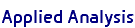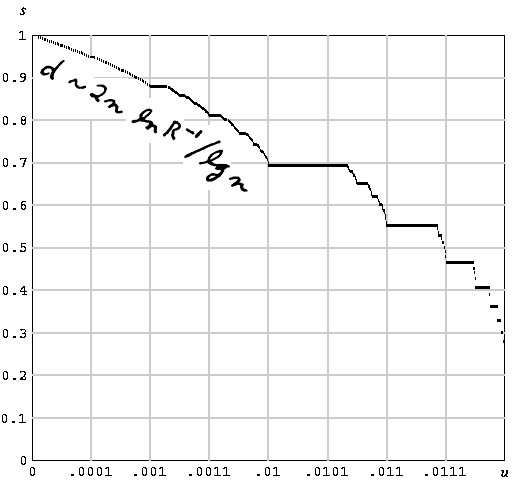Home / Research Interests / Applied Analysis Home Research Interests   Algorithms   Applied Analysis   Games   Complexity   Computer Architecture   Finance   Business   Information Theory Philanthropy Thesis Students Awards Board Memberships K-12 Outreach Education / Employment Biographies Site MapApplied AnalysisA class of error-correcting codes invented by Bose, Chaudhuri, and Hocquenghem became popular in the early 1960s. One can find a primitive binary BCH code of any desired length of the form n = 2m - 1, and any desired designed distance d. The dimension of this code, k, is not easy to determine, even in the asymptotic limit when d and n go to infinity in a fixed ratio, u = d/n. Henry Mann first investigated this enumerative combinatorial problem and solved it for values of the form u = 2-k. Berlekamp solved it for all values of u, and showed that k ~ ns(u), where s(u) is the singular function shown in the figure. For all values of u in the open interval between 0 and 1, it is defined by some interesting manipulations on the binary expansion of u. Quite in addition to its surprising appearance as the solution to an applied problem, the singular function s(u) has some unusual mathematical properties: The Hausdorf dimension of the set of points in the subinterval (a, b) at which the function is not differentiable is s(a). However, the derivative s'(0) turns out to be -1/ln 4, and this fact is crucial to answering another important question: if one requires that k and n approach infinity in a fixed ratio R = k/n, then the distance of the corresponding BCH code is d ~ 2n (ln R-1) / lg n. Technicalities proliferate because one can define the distance in at least three slightly different ways: the design distance, the Bose distance, or the actual distance. The answers differ only in higher order terms. Algebraic Coding Theory, McGraw-Hill, 1968; revised edition, Aegean Park Press, Laguna Hills, CA, 1984. See Chapter 12. "Long Primitive Binary BCH Codes Have Distance d ~ 2n ln R-1/ln n ...", IEEE Trans Inform Theory, IT18:415-426 (1972); Math. Rev. 48-13447.